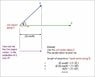# Vector Projection

Hello,

I was wondering, why is the vector projection useful in the way that it is presented? Why isn't just the vector times cosθ sufficient to find the projection of a vector onto another one, why the dot product divided by the magnitude of the vector squared times that same vector?

The book didn't give too much insight to its usefulness other than saying ''it's important'', so it left me a little bit cliffhanging, so just looking for some clarification.

Thanks

Hey Woopydalan.

All projections must satisfy P^2 = P: in other words applying the same projection twice doesn't change the result.

This is probably the best intuitive reason (at least the one I can think of) why it makes sense to define it that way.

Apart from that you gave the geometric interpretation of the projection which finds the component of one vector with respect to another and you can think of projections in terms of orthogonal ones to get an idea of how this naturally ties in with vector decomposition and re-composition.

You should take a look at Gram-Schmidt processes and orthogonal systems to get a better idea of what I'm talking about.

Probably the best way though in the end to think about it, is to think about the idea that you are relating one vector to another in terms of both the distance and the angle between them.

The projection formula achieves both goals in a uniform way: it relates two vectors together taking into account angle and distance of the two vectors maintaining the criteria for a mathematical projection which has P^2 = P (i.e. apply projection again and the result doesn't change).

Projections are useful because of the above in the way that they guarantee that projections "stick" and don't act like functions that would cause them not to "stick".

The visual analog that is nicer is 3D projection where you project an image in 3D space onto a sheet in 2D: you would expect that projecting the image onto itself wouldn't change the image and it doesn't because of this P^2 = P property.

Hopefully this has given you some insight.

Vargo
I could not quite understand the original question. You are asking why express the projection in terms of the dot product instead of the more directly geometric formula involving cosine?

The reason for this is that it is handy to have a formula that you can easily calculate just with vector components. It is purely algebraic. So if you know the lengths of the vectors and you know the angle between them, you can use the more geometric formula you described. But in most cases you just know their components so the formula with the dot product is the most efficient way to do it.

Mandelbroth
Hello,

I was wondering, why is the vector projection useful in the way that it is presented? Why isn't just the vector times cosθ sufficient to find the projection of a vector onto another one, why the dot product divided by the magnitude of the vector squared times that same vector?

The book didn't give too much insight to its usefulness other than saying ''it's important'', so it left me a little bit cliffhanging, so just looking for some clarification.

Thanks
Why wouldn't it be? All math is entertaining. I don't know why, but I'm in a good mood today.Getting back to the point, vector projection is useful in that it can be used in various forms of maths and, perhaps more prominently, physics. I think the most self-evident example of this is in the calculation of "work". Conventionally, work is seen as the product of the magnitudes of force and distance if the force and linear distance vectors are in the same direction. However, the general formula for work, given a distance vector $\vec{PQ}$, is

$W = \left\|proj_{\vec{PQ}}\vec{F}\right\| \left\|\vec{F}\right\|$.

Robert1986
To add to what Chiro has said, relating the projection to the dot product also let's us very easily calculate the projection matrix that will do this for us. So, let's write the dot product of two vectors like this: $\langle v,w \rangle$. Let's say that $v$ is a unit vector and we want to project $w$ onto it. Then we have that the projection is equal to $\langle w,v \rangle w = v\langle w,v \rangle = v(v^*w) = (vv^*)w$ and so the projection matrix is $vv^*$. More generally, if we have some subspace spanned by the orthonormal set $v_1, \ldots , v_k$ the projection matrix onto this set is given by: $P=v_1v_1^* + \cdots + v_kv_k^*$ and you can check that, as Chiro said, $P^2 = P$.

Edgardo
In case you are asking for the derivation of the formula I've drawn a sketch (see attachment). You can see that it is indeed just expressing the cosine in terms of the dot product.

#### Attachments

•projection.jpg
24.6 KB · Views: 1,825
Edgardo
The purple vector is the projection of $\vec{u}$ onto $\vec{v}$.
Now, you have to think of how the purple vector is defined. It has (i) a length and (ii) a direction.

(i) The length of the purple vector is $| \vec{u}| \text{cos}(\theta)$.
(ii) The direction of the purple vector is the same as that of $\vec{v}$.
In order to give the purple vector the length in (i) we normalize $\vec{v}$, see the red vector.
(The red vector is given by $\vec{v}/|\vec{v}|$)

Why can't yo just say the length of the purple vector is ucos(theta) and call it a day? Why the normalizing of v? (what does normalize mean?)

Why can't yo just say the length of the purple vector is ucos(theta) and call it a day? )

You can do that if you happen to know u and theta. However, suppose you are asked to find the projection of the vector (1,2,4) on the vector (5,4,1).

yes, but why are you able to equate ucosθ to ucosθ * (v/IvI)? how can they be equal